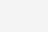# python希尔排序的用法1、根据相邻元素进行排序，如果直接插入排序为步长为1，那么希尔排序就是先按步长为K插入排序。

2、然后在步长K排序的基础上对步长m进行排序，K大于m，最后对步长1进行排序。

```def shell_sort(data_list):
'''
思想：分治策略
使用 for 循环
'''
length = len(data_list)
space  = length//2
while space > 0:
for i in range(space,length ): #默认第一个位置的元素是已排序区间，因此下标从 1 开始
tmp = data_list[i] #待插入的数据
index = i
for j in range(i-space,-1,-space): #从已排序区间查找插入位置
if tmp < data_list[j]:
data_list[j+space] = data_list[j]  #元素向后移动，腾出插入位置
index = j #最后的j即为插入的位置
else:
break
data_list[index] = tmp #插入操作
print(data_list)
space = space // 2
return data_list

def shell_sort2(data_list):
'''
思想：分治策略
使用 while 循环
'''
length = len(data_list)
space  = length//2
while space > 0:
i = space
while i < length: #默认第一个位置的元素是已排序区间，因此下标从 1 开始
tmp = data_list[i] #待插入的数据
j = i
while j >= space and data_list[j - space] > tmp: #从已排序区间查找插入位置
data_list[j] = data_list[j-space]  #元素向后移动，腾出插入位置
j -= space
data_list[j] = tmp #插入操作
print(data_list)
i +=1
space = space // 2
return data_list```